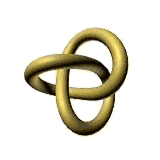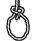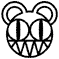# INTRODUCTIONThis paper will describe different qualities that are consistent in equivalent knots.  Since the main goal of knot theory is to prove equivalence between two given knots, the qualities discussed, called invariants, are very important in knot theory.  I will begin by providing the mathematical definition of a knot, and explaining what it means for two knots to be equivalent.  Then I will proceed into a discussion of invariants.  The paper will conclude with an explanation of the composition of knots.Everyone knows what a knot is in ordinary layman's terms.  A piece of string is knotted if it is twisted around itself in some fashion.The mathematical definition is a little more specific than this.  If the knotted piece of string is then taped together at the two ends, it satisfies the definition of a mathematical knot.  In his paper called "The Theory of Knots", Lee Neuwirth defines a mathematical knot more precisely: a knot is a one-dimensional closed curve in three dimensional space without self-intersections.  By closed, we mean that the curve begins and ends at the same point.The simplest knot is called the unknot, or the trivial knot. It has no twists or crossings. The unknot can be distorted deceptively to look like a more complicated knot. UNKNOT

The Unknot

A mathematical definition for equivalence is necessary to begin the description of invariants.  Two knots are equivalent when they can be pulled, pushed, stretched, or twisted until they look the same.  This intuitive definition will suffice for someone with a limited background in mathematics.  However, there is a precise mathematical definition for this. I borrow it from Kunio Murasugi's book, Knot Theory & It's Applications, and include it here.kMain menu      1. History               2.Intro               3.Invariants     4.Composition   5.Conclusion